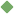parameter 1. [ noun ] (mathematics) a constant in the equation of a curve that can be varied to yield a family of similar curves Synonyms: parametric_quantity Related terms: constant degree_of_freedom To share this definition press "text" (Facebook, Twitter) or "link" (blog, mail) then paste text link 2. [ noun ] any factor that defines a system and determines (or limits) its performance Related terms: factor 3. [ noun ] (mathematics,statistics) a quantity (such as the mean or variance) that characterizes a statistical population and that can be estimated by calculations from sample data Related terms: quantity Similar spelling: Parmeter   parametric   parametritis   paramedic   paramedical